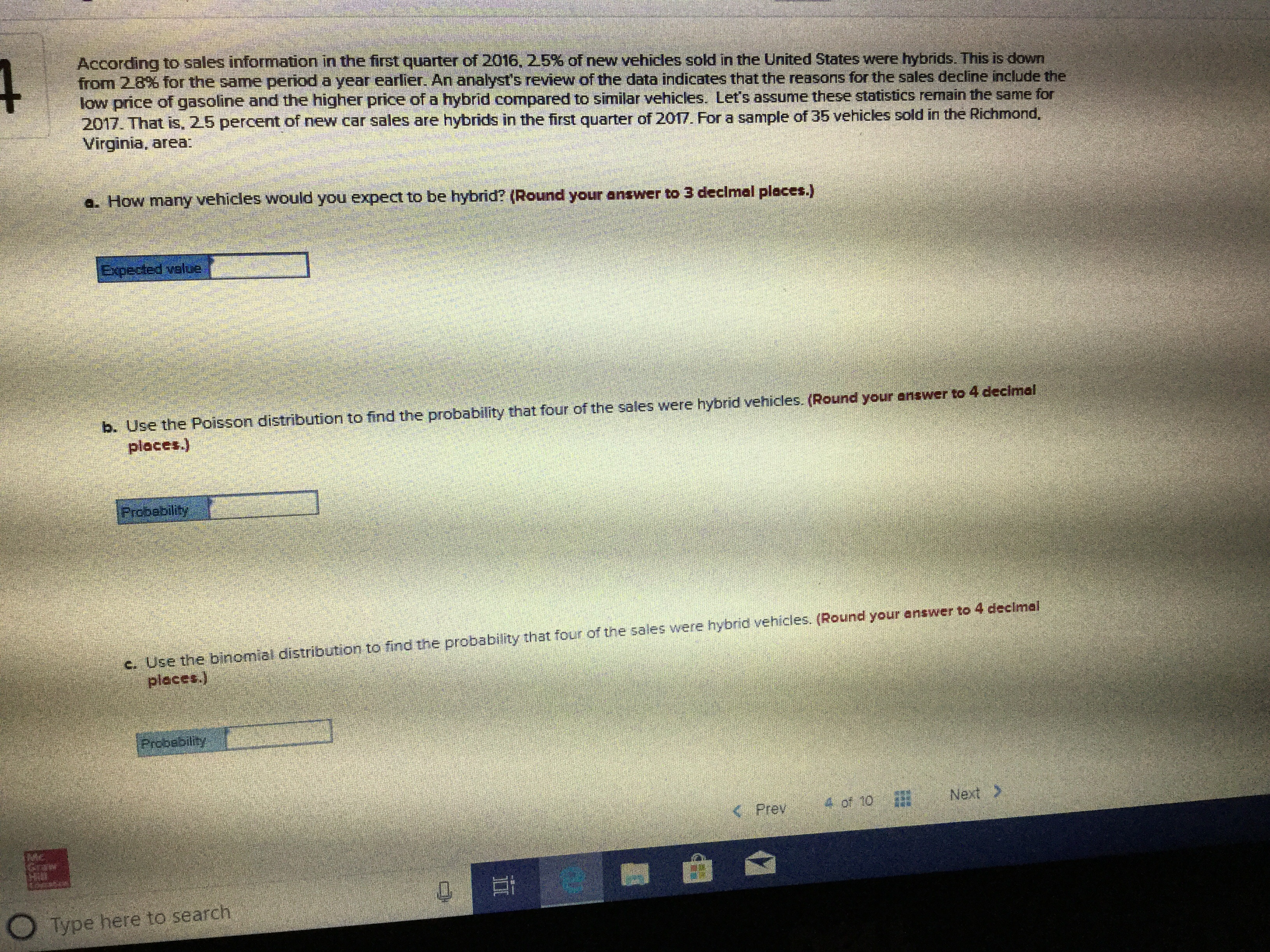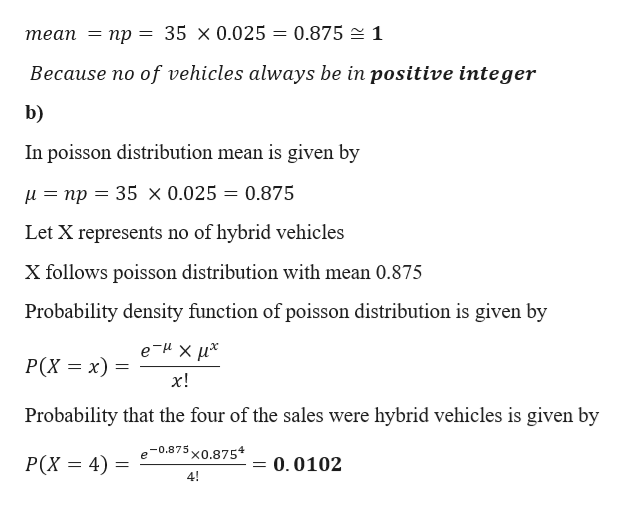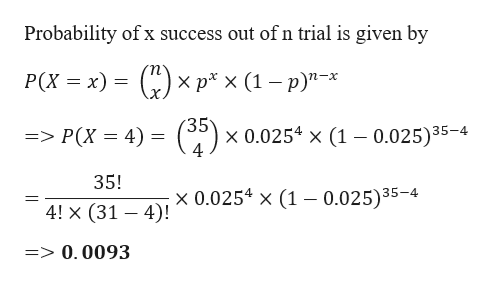# 4According to sales information in the first quarter of 2016, 2.5% of new vehicles sold in the United States were hybrids. This is downfrom 2.8% for the same period a year earlier. An analyst's review of the data indicates that the reasons for the sales decline include thelow price of gasoline and the higher price of a hybrid compared to similar vehicles. Let's assume these statistics remain the same for2017. That is, 2.5 percent of new car sales are hybrids in the first quarter of 2017. For a sample of 35 vehicles sold in the Richmond,Virginia, area:a. How many vehicles would you expect to be hybrid? (Round your answer to 3 decimal places.)Expected veloeb. Use the Poisson distribution to find the probability that four of the sales were hybrid vehicles. (Round your answer to 4 decimalplaces.)Probebilityc. Use the binomial distribution to find the probability that four of the sales were hybrid vehicles. (Round your answer to 4 decimelplaces.)ProbebilityNext4 of 10

Question
251 viewshelp_outlineImage Transcriptionclose4 According to sales information in the first quarter of 2016, 2.5% of new vehicles sold in the United States were hybrids. This is down from 2.8% for the same period a year earlier. An analyst's review of the data indicates that the reasons for the sales decline include the low price of gasoline and the higher price of a hybrid compared to similar vehicles. Let's assume these statistics remain the same for 2017. That is, 2.5 percent of new car sales are hybrids in the first quarter of 2017. For a sample of 35 vehicles sold in the Richmond, Virginia, area: a. How many vehicles would you expect to be hybrid? (Round your answer to 3 decimal places.) Expected veloe b. Use the Poisson distribution to find the probability that four of the sales were hybrid vehicles. (Round your answer to 4 decimal places.) Probebility c. Use the binomial distribution to find the probability that four of the sales were hybrid vehicles. (Round your answer to 4 decimel places.) Probebility Next 4 of 10
check_circle

Step 1

Given data

No of vehicles (n) = 35

Probability that the new car sales is hybrid (p)  = 0.025

a)

Expected no of vehicles would be hybrid is given byhelp_outlineImage Transcriptioncloseтеап — пр — 35 х 0.025 - 0.875 1 Because no of vehicles always be in positive integer b) In poisson distribution mean is given by 35 x 0.025 = 0.875 =np Let X represents no of hybrid vehicles X follows poisson distribution with mean 0.875 Probability density function of poisson distribution is given by eиxих Р(х %3D х) х! Probability that the four of the sales were hybrid vehicles is given by -0.875 e 'x0.8754 Р(x %3D4) = 0.0102 4! fullscreen
Step 2

c)

Now using binomial distribution

Probability that the four of the sales were hybrid vehicles is given by

Applyin...help_outlineImage TranscriptioncloseProbability of x success out ofn trial is given by Р(X %3D х) (К)x р*x (1—р)"-х = > P(X 4) )> x 0.0254 x (1 0.025)35-4 = 35! x 0.0254 x (1 0.025)35-4 4! x (31 4) => 0.0093 fullscreen

### Want to see the full answer?

See Solution

#### Want to see this answer and more?

Solutions are written by subject experts who are available 24/7. Questions are typically answered within 1 hour.*

See Solution
*Response times may vary by subject and question.
Tagged in
MathStatistics

### Other